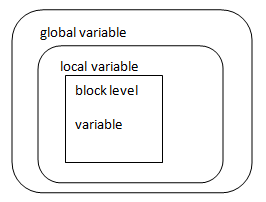Types of variables in C++
Ratings:
(4)
Views:717## C++ Local Variables

The variable declared inside function is called local variable in C++

• Memory for this variable is allocated on execution of function and after completion of function
• The scope of this variable within function
• Local variable are called auto variable.
• Auto is storage class and variable is never declared without class
• Storage class define scope life time and storage of variable.

Example #include<iostream.h> void main() { int x; int Y; int Z; Cout << X << endl; cout<<Z<<endl; } o/p : The output of above program is garbage value, Because local variables are not given any default values.

• C++ allows to declare local variable anywhere within function

Example # include <iostream.h> void main() { int n1; cout<< “\n input n1 value”; cin >> n1; int n2; cout<< “\n input n2 value”; cin>> n2; int n3; n3=n1+n2; cout<< “\n sum is ” << n3 } os void main() {

• C++ allows to declare block level variables.

## C++ Block level variables

The variables which are declared inside the anyone of the following block is called as Block level variables in C++

1. Anonymous block
2. Conditional block
3. Iterational block

Example if(<condition>) { int x;    - > block level } Conditional Block while(<condition>) { int y;          Block level } Iterational block Example # include <iostream.h void main() { char name; int sub1,sub2; cout<<”\n input name”; cin>> name; cout<< “\n input two subjects”; cin>> sub1 >> sub2; cout<< “\n input two subjects”; cin>> sub 1>> sub 2; cout << “\n name” << name; if(sub1>=40 && sub2 >=40) { int total = sub1+sub2; flat avg=total/3; cout<<”\n Total” << total; cout<< “\n Avg” << avg; cout<< “\n Result is pass”; } else cout<< “\n result fail” }

• C++ allows to declare local and block level variables with same name
• This is called as hicling

### Program

void main () { int x=10; int y=20; cout<<”\n local variable x=”<<x    10 cout<<”\n local variable y=”<<y; 20 { int z=30; cout<< “\n Block local variable z=” <<Z; 30 int x=40; cout<<”\n Block local variable=” <<x; cout<<”\n block variable y= <<y”; } }

• we can’t access local variable within Block

## C++ Global Variables

• The variable declare outside the function is called global variable in C++
• Variable is declared at global to share data between one or more than one function
• These are called external variables.
• Extern is a storage class

Syntax : int x; - > global variable void main() { int y; - > local variable if(<condition>) { int z; block level variable }• global variables are given default value
• if global variable is int, double, float then default value is zero.
• c++ allows to declare local and global variable with same name.
• used in c++ 1) ::  (scope resolution)
• pointers belongs to c++ 2) * (pointer to member access)

3  *(pointer to pointer member access operator)

• Why we used scope resolution operator ?
• It is a binary operator and it also work as unary operator